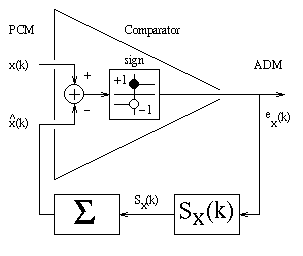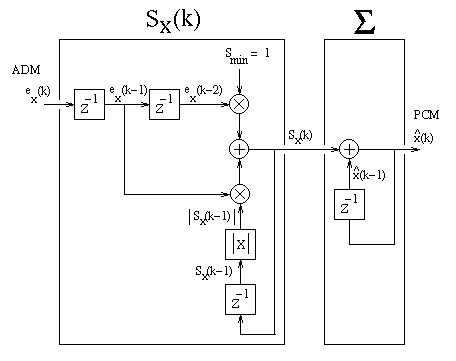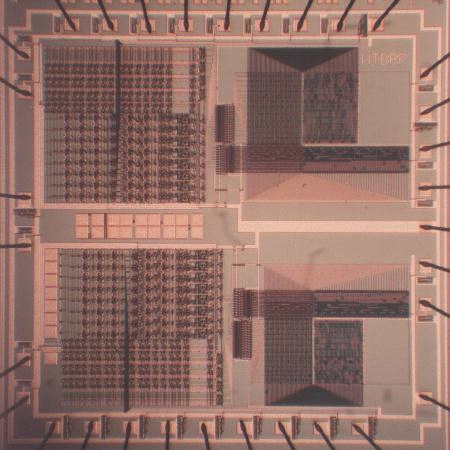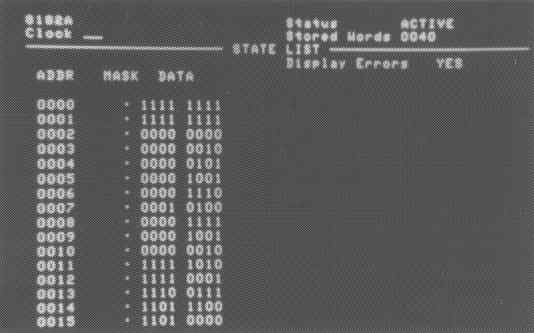# Brent Petersen - M.A.Sc. Summary

## Abstract

A silicon compiler is a computer program that generates IC layouts from a high-level specification. In the SPIL silicon compiler a digital signal processing algorithm is specified in a language similar to Pascal, and IC layouts are generated using a simple register-transfer architecture with a data path controlled by a finite state machine. For a silicon compiler to be an effective design automation tool it is essential to include some type of performance evaluation to estimate power dissipation, layout area and propagation delays of the generated chip layout.

A design aid called EPAD is used for performance estimation of propagation delays, power dissipation and silicon areas of CMOS VLSI circuits. The objective is to provide the designer with an analysis of the IC layout. The designer can use this analysis to change the algorithm or intermediate levels of silicon compilation.

SPIL and EPAD are used in the design of a chip which compresses and decompresses speech by performing conversions between pulse code modulation and adaptive delta modulation. The algorithms for these conversions were written in the SPIL language, and layouts were generated and combined into one coder-decoder (codec) chip. An EPAD analysis was performed on this chip. Simulation files obtained from EPAD made it possible to identify possible design errors and to predict the maximum operating frequency. This was followed by fabrication and testing of the chip. Test results on fabricated chips compare favourably to EPAD predictions. The results made it possible to evaluate the effectiveness of the silicon compiler and to calibrate the performance evaluator.

The goal of this research is to show that the state of the art is advanced enough that a chip can be efficiently designed using SPIL with EPAD and that the chip can satisfy the requirements of specific applications.

## Summary

### The transmitter

The following is the signal flow graph of the pulse code modulation to adaptive delta modulation conversion, the transmitter.By examining the signal flow graph of the transmitter, the following program was written in the language of the compiler, SPIL (Simplified Pascal Into Layout).
```PROGRAM PCM_to_ADM ;

CONST
_data_width = 8 ;

VAR
PCM_input  : input_port  CONNECT      DOWNWARD ;
ADM_output : output_port CONNECT 0..0   UPWARD ;

Ex      ,        { Starting from LSB(0) : Ex(k-1), Ex(k-2) }
Sx_of_k ,                     { Step to next predicted PCM }
X_of_k  : integer ;                      { Last PCM output }

PROCEDURE _reset ;             { Chip initialization procedure }
BEGIN
Ex      := 0 ;                { Make Ex(k-1) = Ex(k-2) = 0 }
X_of_k  := 0 ;
Sx_of_k := 0 ;
END ;

BEGIN
IF Sx_of_k < 0    THEN Sx_of_k := 0 - Sx_of_k ;

IF Ex = ???????0B THEN Sx_of_k := 0 - Sx_of_k ;

_add_in_1 := Sx_of_k ;          { IF Ex(k-2) = 1 THEN       }
IF Ex = ??????1?B THEN          {                           }
_add_in_2 :=  1              {    Sx_of_k := Sx_of_k + 1 }
ELSE                            { ELSE                      }
_add_in_2 := -1 ;            {    Sx_of_k := Sx_of_k - 1;}
Sx_of_k := _add_out ;           { (No over/under-flow check)}

X_of_k := X_of_k + Sx_of_k ;    {  No over/under-flow check }

Ex := Ex << 1 ;   { Shift signals left   i.e. one time step }

IF PCM_input > X_of_k THEN Ex := Ex + 1 ;

END.         { PCM_input must have remained valid all the time }
```

The following is the signal flow graph of the adaptive delta modulation to pulse code modulation conversion, the receiver.The following is the program for the receiver.
```PROGRAM ADM_to_PCM ;

CONST
_data_width = 8 ;

VAR
ADM_input  : input_port  CONNECT 0..0   UPWARD ;
PCM_output : output_port CONNECT      DOWNWARD ;

Ex      ,        { Starting from LSB(0) : Ex(k-1), Ex(k-2) }
Sx_of_k ,                     { Step to next predicted PCM }
X_of_k  : integer ;                      { Last PCM output }

PROCEDURE _reset ;             { Chip initialization procedure }
BEGIN
Ex      := 0 ;                { Make Ex(k-1) = Ex(k-2) = 0 }
X_of_k  := 0 ;
Sx_of_k := 0 ;
END ;

BEGIN
IF Sx_of_k < 0    THEN Sx_of_k := 0 - Sx_of_k ;

IF Ex = ???????0B THEN Sx_of_k := 0 - Sx_of_k ;

_add_in_1 := Sx_of_k ;          { IF Ex(k-2) = 1 THEN       }
IF Ex = ??????1?B THEN          {                           }
_add_in_2 :=  1              {    Sx_of_k := Sx_of_k + 1 }
ELSE                            { ELSE                      }
_add_in_2 := -1 ;            {    Sx_of_k := Sx_of_k - 1;}
Sx_of_k := _add_out ;           { (No over/under-flow check)}

X_of_k := X_of_k + Sx_of_k ;    {  No over/under-flow check }

Ex := Ex << 1 ;   { Shift signals left   i.e. one time step }
IF ADM_input = ???????1B THEN EX := Ex + 1 ;

PCM_output := X_of_k ;
END.         { ADM_input must have remained valid all the time }
```

### The chip

It took two months to become familiar with the SPIL design environment and the receiver was designed during this time. After familiarity with design environment, the transmitter only took 9 days to take from the idea to the final layout.

The top half is the transmitter, the bottom is the receiver. The right half contains the two finite state machines. The left half contains the two data paths and address decoder logic. The transmitter and receiver operate independently and only share the power rails, 5 volts and ground. The 8-bit data paths run horizontally and can be seen in the picture. In the receiver, the data path is above the address decoder logic. In the transmitter, the data path is below the address decoder logic.• fabricated using a 3-micron CMOS process through the CMC and Northern Telecom
• is 4.511mm by 4.511mm
• mean clock frequencies averaged over 5 fabricated chips are 3.11 MHz for the transmitter and 4.27 MHz for the receiver (which is greater than the 1.088 MHz required to process 8-bit 8-KHz pulse code modulation)
• mean power dissipation is 31mW at 1 MHz clock frequencies
• finite state machines: transmitter 38 states, receiver 33 states
• confirmed to work using a test pattern with 80.1% single-stuck-at fault coverage.
• picture taken in an ad hoc manner using a Hasselblad camera on a tripod aimed through one eye piece of a CMC probe station. (distortion at corners and a fibre present in the middle of the picture)

### The data analyser output

The following is one output from the screen of the HP 8182A data analyser. It shows the 8-bit PCM output of the receiver in response to an ADM input that is 1 up to ADDR 0007. In base ten, these outputs, up to and including ADDR 0007, are -1, -1, 0, 2, 5, 9, 14, 20. This image was the first proof that the chip worked.This page is located at `http://www.ece.unb.ca/petersen/pubs/theses/MASc/`